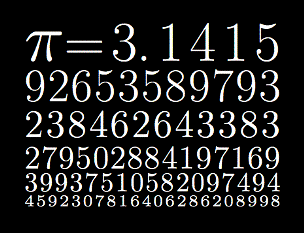# Naming decimal places

Naming decimal places are another way of representing rational numbers, besides fractions. A decimal number contains a decimal point and it is based on the number 10. The value of a digit is determined by its position relative to the decimal point. On the left side of the decimal point are integers, whose value ranges from negative infinity to infinity. On the right side of the decimal point, the value of the number is always between 0 and 1.
Example: In the number 17.421, the value left from the decimal point (17) represents the whole number or the integer, while .421 represents a value that is larger than 0 and smaller than 1.The value .421 can also be represented by the fraction 421/1000 or an equivalent of that fraction.The position of the digits in relation to the decimal point is crucial, as it determines the value of the each digit in the number. The further to the right from the decimal point we go, the places represent a smaller value. Since the decimal system is based on the number 10, the first place behind the decimal point represents tenths, the second place represents hundredths and so on.

Example: The number 1743.465 can be written down as 1*1000 + 7*100 + 4*10 + 3*1 + 4*1/10 + 6*1/100 + 5*1/1000. The number behind the decimal point can be read as 4 tenths, 6 hundredths and 5 thousandths or 465 thousandths. The same principle applies, no matter how many places behind the decimal point there are in a number. If there are 4 places behind the decimal point, we are talking about ten thousandths (cialis online). If there are 5 places behind the decimal point, then we are talking about hundred thousandths and so on. Naming decimal places is easy, you just need to catch the rhythm.

## Naming decimal places exams for teachers

 Exam Name File Size Downloads Upload date Naming decimal places – very easy 149.6 kB 4169 September 3, 2019 Naming decimal places – easy 146.2 kB 3268 September 3, 2019 Naming decimal places – medium 164.7 kB 4081 September 3, 2019 Naming decimal places – hard 169 kB 3259 September 3, 2019 Naming decimal places – very hard 164.9 kB 2938 September 3, 2019